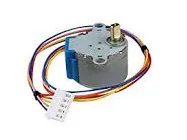Project tutorial# Getting Started With Stepper Motor 28BYJ-48 © LGPL

The basics of how to control a stepper motor

• 37,203 views
• 11 respects

## Apps and online services

This project is an easy introduction to stepper motors and aims to introduce null-level beginners to stepper motors and their respective drivers.

This project used 28byj-48 stepper motor and UNL-2003 stepper motor driver.

This project is based on the arduino UNO microprocessor.

### Schematic of circuit

Follow the schematic given above. The connections are:

Stepper Motor :

Usually the female header pins of the motor are grouped and connected to the corresponding port on the uln-2003 but there can be exceptions, follow the diagram above. (view enlarged, it will help a lot!)

Arduino and Driver:

Any digital pins of your choice can be connected to the driver, starting from left for Coil A, B, C, D There are pins for E, F and G too, but we will not be using those for this project.

Connect the + port to the 5v port of the arduino, (if you wish you can also connect to the 3.3v) and the - port to the GND port of the arduino. (There are multiple GND ports, select any one.)

### How the Stepper motor works

The stepper motor works by applying pulses of current respectively to each of the coils of the motor which each move the stepper motor one small step. Using this sequence repeatedly we generate movement which can be monitored to incredible levels of accuracy without PWM outputs.

Here we use the eight-phase model which energizes each coil respectively sometimes two at once. There is no need for PWM outputs here as the pulses are digital 1 or 0.

The order in which we apply the power affects the direction of the motor. If we apply power sequentially from A to D the motor turns clockwise while if we apply power sequentially from D to A the motor turns anti-clockwise.

### Code

``````#define A 2
#define B 3
#define C 4
#define D 5
#define NUMBER_OF_STEPS_PER_REV 512
``````

This segment defines the pins connected to each coil.

We are using 512 steps per revolution for this stepper motor.

Change this number to whatever your one is of.

``````void setup(){
pinMode(A,OUTPUT);
pinMode(B,OUTPUT);
pinMode(C,OUTPUT);
pinMode(D,OUTPUT);
}
``````

This segment initializes each pin as output pin.

``````void write(int a,int b,int c,int d){
digitalWrite(A,a);
digitalWrite(B,b);
digitalWrite(C,c);
digitalWrite(D,d);
}

void onestep(){
write(1,0,0,0);
delay(5);
write(1,1,0,0);
delay(5);
write(0,1,0,0);
delay(5);
write(0,1,1,0);
delay(5);
write(0,0,1,0);
delay(5);
write(0,0,1,1);
delay(5);
write(0,0,0,1);
delay(5);
write(1,0,0,1);
delay(5);
}
``````

This segment does all the actual work. The onestep function energizes each coil in turn to produce movement. The delays in between determine the speed of onestep.

``````void loop(){
int i;
i=0;
while(i<NUMBER_OF_STEPS_PER_REV){
onestep();
i++;
}
``````

This segment will turn the motor one full turn (clockwise).

## Code

##### CodeArduino
```#define A 2
#define B 3
#define C 4
#define D 5

#define NUMBER_OF_STEPS_PER_REV 512

void setup(){
pinMode(A,OUTPUT);
pinMode(B,OUTPUT);
pinMode(C,OUTPUT);
pinMode(D,OUTPUT);
}

void write(int a,int b,int c,int d){
digitalWrite(A,a);
digitalWrite(B,b);
digitalWrite(C,c);
digitalWrite(D,d);
}

void onestep(){
write(1,0,0,0);
delay(5);
write(1,1,0,0);
delay(5);
write(0,1,0,0);
delay(5);
write(0,1,1,0);
delay(5);
write(0,0,1,0);
delay(5);
write(0,0,1,1);
delay(5);
write(0,0,0,1);
delay(5);
write(1,0,0,1);
delay(5);
}

void loop(){
int i;
i=0;
while(i<NUMBER_OF_STEPS_PER_REV){
onestep();
i++;
}
```

#### Author##### debanshudas23
• 3 projects
• 7 followers

• Image of stepper motor by Amazon.in

June 1, 2020

#### Members who respect this project

See similar projects
you might like

#### Driving 28BYJ-48 Stepper Motor Control with Joystick

Project tutorial by BEASTIDREES62

• 10,367 views
• 19 respects

• 42,379 views
• 48 respects

• 26,854 views
• 52 respects

#### Large Stepper Motor Control A4988

Project tutorial by 3 developers

• 14,090 views
• 12 respects

#### Unipolar Stepper Motor Controlling Library

Project tutorial by ambhatt

• 13,036 views